Next: Linear observations Up: The derivation of the Previous: The derivation of the

The Derivation

We want to derive an optimal estimate,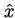, of a field, x, as a linear combination of observation data,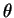,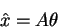(1)

Our goal is to derive the form of the matrix, A, so that the expected mean square difference between the estimated field and the actual field ( x ) is minimized,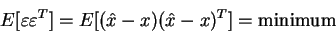(2)

If we put (1) into (2) and expand, we get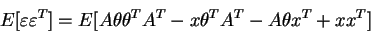(3)

If we let Cx be the autocorrelation of the field ( E[x xT] ),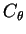be the autocorrelation of the observations (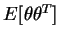), and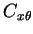be the cross correlation between the field and the observations (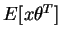), then we can write the above as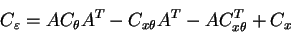(4)

The next step requires the application of the following matrix identity (proved in the appendix),

 (A - B C-1) C (A - B C-1)T - B C-1 BT = A C AT - B AT - (B AT)T (5)

using A in (4) for A in (5), andfor B as well asfor C, we can reduce (x) to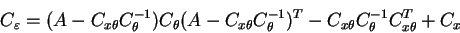(6)

(note we have also used the fact that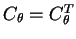).

The matrices, is an autocorrelation matrix therefore both it and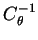are nonnegative definite (see appendix), therefore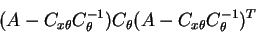(7)

and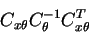(8)

are both matrices with positive diagonal elements. This means that the diagonal elements of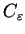are therefore minimized when it is true that,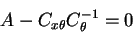(9)

Therefore we have,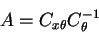(10)

This is the estimator that we are seeking.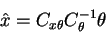(11)

Further, we can write down what the expected error for the estimator as,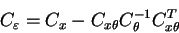(12)

Equations (11) and (12) constitute the Gauss-Markov estimator for the linear minimum means square estimate of a random variable.Next: Linear observations Up: The derivation of the Previous: The derivation of the
Skip Carter
1999-12-08